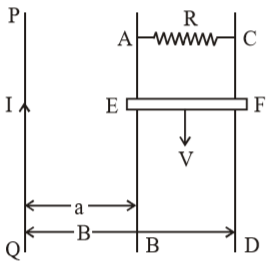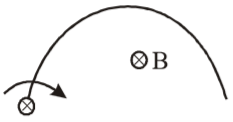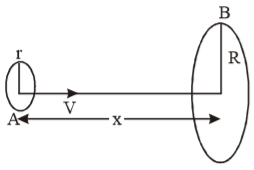A wire of fixed lengths is wound on a solenoid of length $\mathcal{l}$ and radius r. Its self inductance is found to be L. Now if same wire is wound on a solenoid of length $\mathcal{l}/2$ and radius r/2, then the self inductance will be –

1.  $2\mathrm{L}$

2.  $\mathrm{L}$

3.  $4\mathrm{L}$

4.  $8\mathrm{L}$

Concept Questions :-

Self-inductance
High Yielding Test Series + Question Bank - NEET 2020

Difficulty Level:

A wire in the form of a circular loop of radius 10 cm lies in a plane normal to a magnetic field of 100 T. If this wire is pulled to take a square shape in the same plane in 0.1 s, average induced emf in the loop is:

1.  6.70 volt

2.  5.80 volt

3.  6.75 volt

4.  5.75 volt

Concept Questions :-

Faraday 's law and lenz law
High Yielding Test Series + Question Bank - NEET 2020

Difficulty Level:

A superconducting loop of radius R has self inductance L. A uniform and constant magnetic field B is applied perpendicular to the plane of the loop. Initially current in this loop is zero. The loop is rotated by 180°. The current in the loop after rotation is equal to –

1. zero

2. $\frac{{\mathrm{B\pi R}}^{2}}{\mathrm{L}}$

3. $\frac{2{\mathrm{B\pi R}}^{2}}{\mathrm{L}}$

4. $\frac{{\mathrm{B\pi R}}^{2}}{2\mathrm{L}}$

Concept Questions :-

Self-inductance
High Yielding Test Series + Question Bank - NEET 2020

Difficulty Level:

A coil of inductance 8.4 mH and resistance 6$\mathrm{\Omega }$ is connected to a 12 V battery. The current in the coil is 1.0 A at approximately the time.

1.  500 ms

2.  20 ms

3.  35 ms

4.  1 ms

Concept Questions :-

LR circuit
High Yielding Test Series + Question Bank - NEET 2020

Difficulty Level:

PQ is an infinite current carrying conductor. AB and CD are smooth conducting rods on which a conductor EF moves with constant velocity V as shown. The force needed to maintain constant speed of EF is –1.

2.

3.

4.

Concept Questions :-

Motional emf
High Yielding Test Series + Question Bank - NEET 2020

Difficulty Level:

A 50 turns circular coil has a radius of 3 cms, it is kept in a magnetic field acting normal to the area of the coil. The magnetic field B increased from 0.10 tesla to 0.35 tesla in 2 milliseconds. The average induced emf in the coil is-

1.  1.77 volts

2.  17.7 volts

3.  177 volts

4.  0.177 volts

Concept Questions :-

Faraday 's law and lenz law
High Yielding Test Series + Question Bank - NEET 2020

Difficulty Level:

A semicircular wire of radius R is rotated with constant angular velocity $\mathrm{\omega }$ about an axis passing through one end and perpendicular to the plane of the wire.There is a uniform magnetic field of strength B. The induced emf between the ends is -

1. ${\mathrm{B\omega R}}^{2}/2$

2. $2{\mathrm{B\omega R}}^{2}$

3. is variable

4. none of these

Concept Questions :-

Motional emf
High Yielding Test Series + Question Bank - NEET 2020

Difficulty Level:

The inductance of a closed-packed coil of 400 turns is 8 mH. A current of 5 mA is passed through it. The magnetic flux through the coil is approximately

1.

2.

3.

4.

Concept Questions :-

Self-inductance
High Yielding Test Series + Question Bank - NEET 2020

Difficulty Level:

Loop A of radius (r << R) moves towards loop B with a constant velocity v in such a way that their planes are always parallel.What is the distance (x) between the two loops when the induced emf in loop A is maximum –

1. $\mathrm{R}$

2. $\frac{\mathrm{R}}{\sqrt{2}}$

3. $\frac{\mathrm{R}}{2}$

4. $\mathrm{R}\left(1-\frac{1}{\sqrt{2}}\right)$

Concept Questions :-

Motional emf
High Yielding Test Series + Question Bank - NEET 2020

Difficulty Level:

The current in an L – R circuit builds up to ${\left(3/4\right)}^{\mathrm{th}}$ of its steady state value in 4 seconds. The time constant of this circuit is

1.

2.

3.

4.

Concept Questions :-

LR circuit